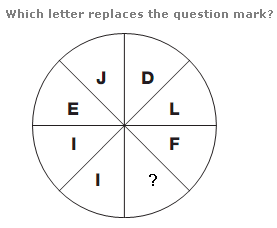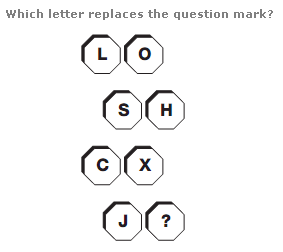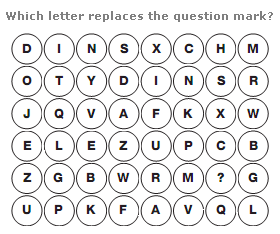# Puzzles - Missing letters puzzles

### Exercise :: Missing letters puzzlesAnswer : K Explanation : Split the circle into 4 quarters, horizontally and vertically, with 2 segments each. Starting with the top left segment, the sum of the numerical values of the letters in the 2 segments equals 15. The sum of the next pair of segments clockwise equals 16, then 17, then 18.Answer : Q Explanation : Taking each pair of letters in each row, the numerical value of one letter equals the inverse numerical value of the other letter.Answer : H Explanation : Start on the top left of the diagram, and move clockwise in a spiral towards the centre. Letters advance through the alphabet in steps of 5.